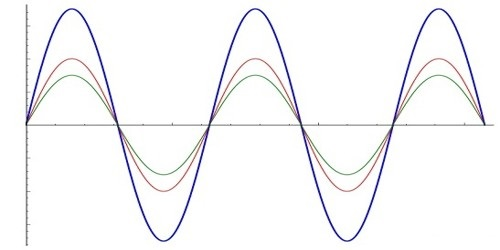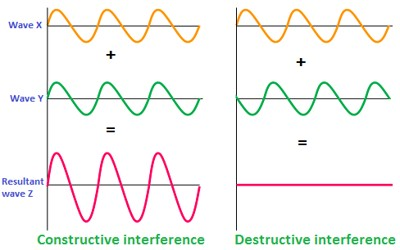Physics

# Interference of LightIf two light-waves having same frequencies and amplitudes emitted from two coherent sources pass through the same point in a medium, the point becomes very bright when the two waves superpose at that point with the same phase and become dark if the two waves superpose with opposite phase. This phenomenon of variation of intensity of light created due to the superposition of light-waves is called interference.

If these two waves are incident at a point with the same phase i.e., if the crests or troughs of those two waves are incidents at that point, the resultant of the amplitude at that point is equal to the summation of the amplitudes of these two waves.

Since the intensity is proportional to the square of the amplitude, hence if these two waves are incident at a point in the same phase, the point will look bright. On the other hand, if these two waves are incident at a point with opposite phase, that means if the crest of one wave and the trough of the other wave are incident at that point, then the resultant amplitude will be zero. As a result, the point will appear dark. This is the interference of light.Interference of light supports the wave theory of light. In 1801, Thomas Young discovered the interference of light. There are two kinds of interference: (i) Constructive interference and (ii) Destructive interference.

Constructive interference: If the bright point is observed due to the superposition of two light waves having the same frequency and amplitude emitting from two sources, then that interference is called constructive interference.

Destructive interference: If a dark point is observed due to the superposition of two light waves having the same frequency and amplitude emitting from two then that interference is called destructive interference.